## Get genotype file

# Get the example bedfile from package bigsnpr
bedfile <- system.file("extdata", "example.bed", package = "bigsnpr")

## Principal Component Analysis

# Half of the cores you have on your computer
NCORES <- nb_cores()
# Exclude Long-Range Linkage Disequilibrium Regions of the human genome
# based on an online table.
ind.excl <- snp_indLRLDR(infos.chr = CHR, infos.pos = POS)
# Use clumping (on the MAF) to keep SNPs weakly correlated with each other.
# See https://privefl.github.io/bigsnpr/articles/pruning-vs-clumping.html
# to know why I prefer using clumping than standard pruning.
ind.keep <- snp_clumping(G, infos.chr = CHR,
exclude = ind.excl,
ncores = NCORES)
# Get the first 10 PCs, corresponding to pruned SNPs
obj.svd <- big_randomSVD(G, fun.scaling = snp_scaleBinom(),
ind.col = ind.keep,
ncores = NCORES)
# As obj.svd has a class and a method plot.
# Scree plot by default
plot(obj.svd)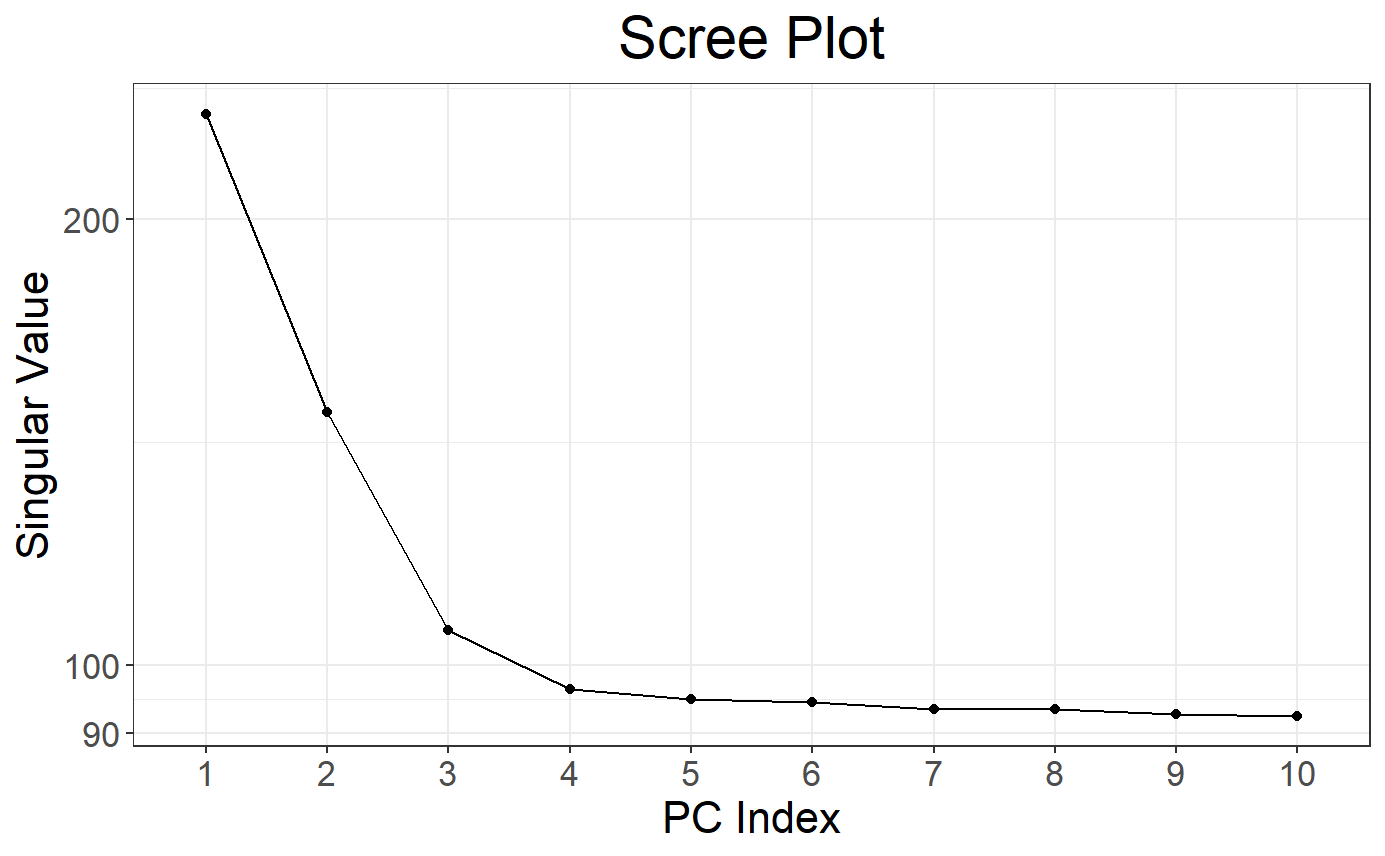# Score (PCs) plot
plot(obj.svd, type = "scores")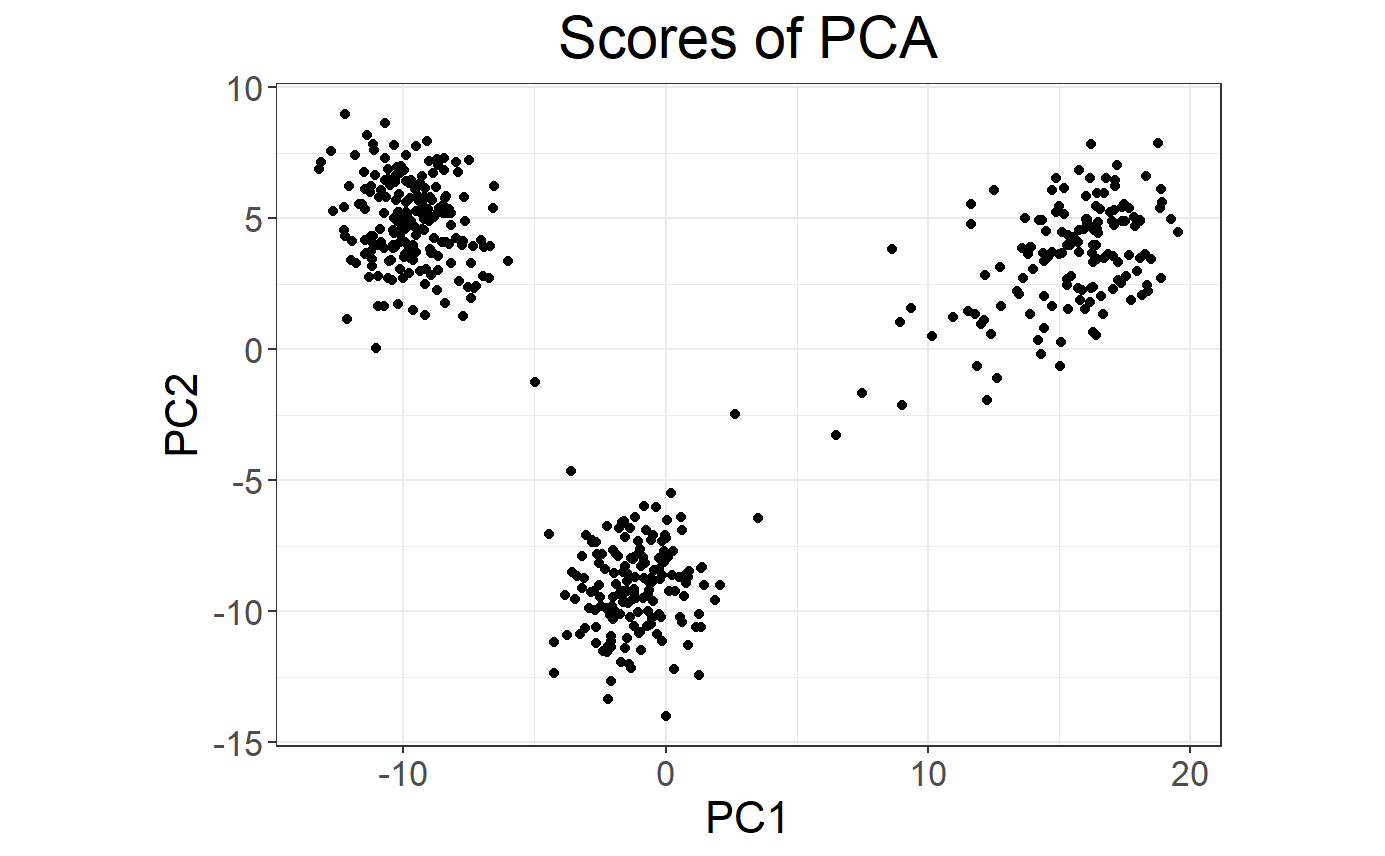# As plot returns an ggplot2 object, you can easily modify it.
# For example, you can add colors based on the population.
library(ggplot2)
## Warning: package 'ggplot2' was built under R version 3.6.3
plot(obj.svd, type = "scores") +
aes(color = pop <- rep(c("POP1", "POP2", "POP3"), c(143, 167, 207))) +
labs(color = "Population")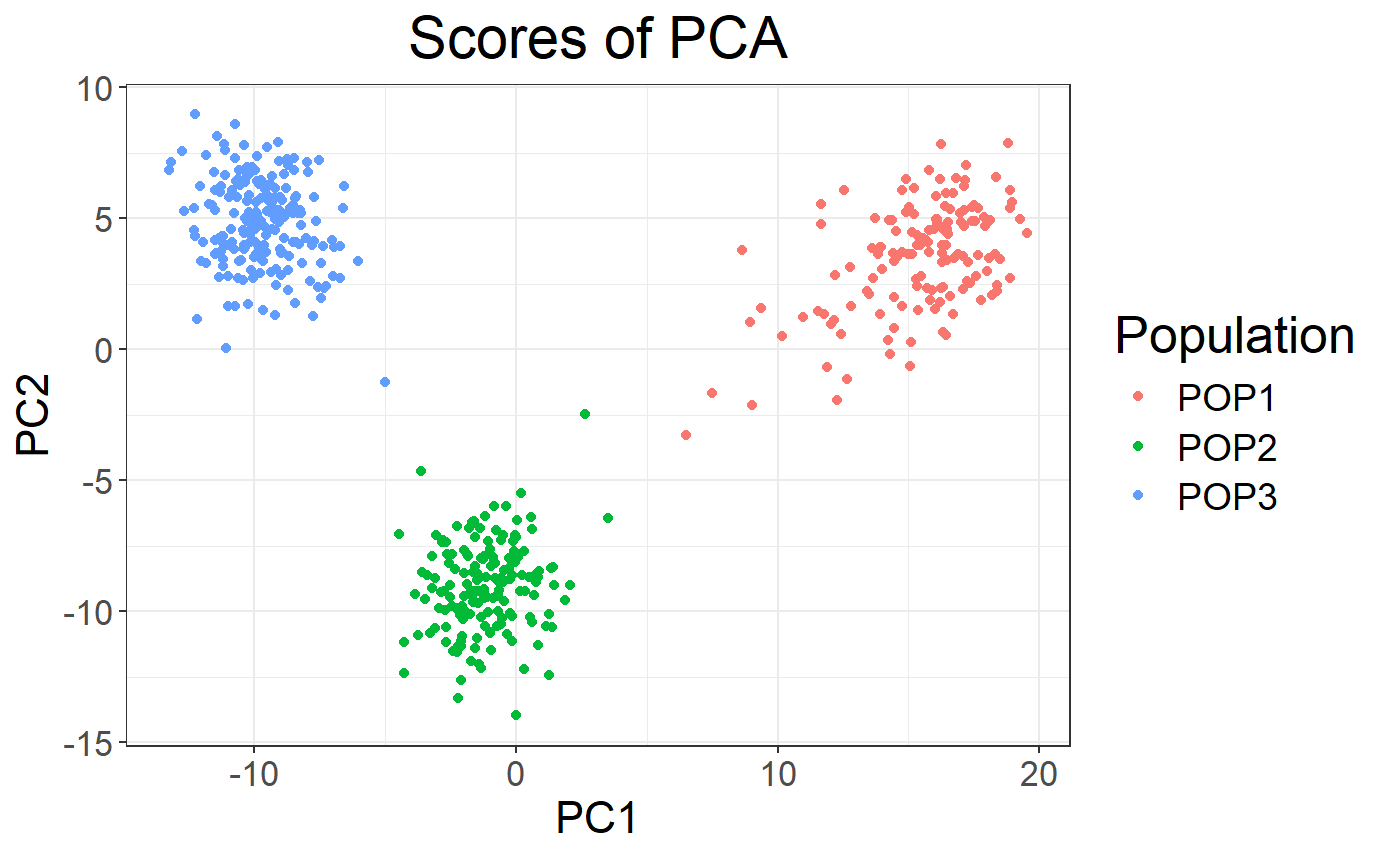## Genome-Wide Association Study

# Fit a logistic model between the phenotype and each SNP separately
# while adding PCs as covariates to each model
y01 <- obj.bigSNP$fam$affection - 1
obj.gwas <- big_univLogReg(G, y01.train = y01,
covar.train = obj.svd$u, ncores = NCORES) # Q-Q plot of the object snp_qq(obj.gwas)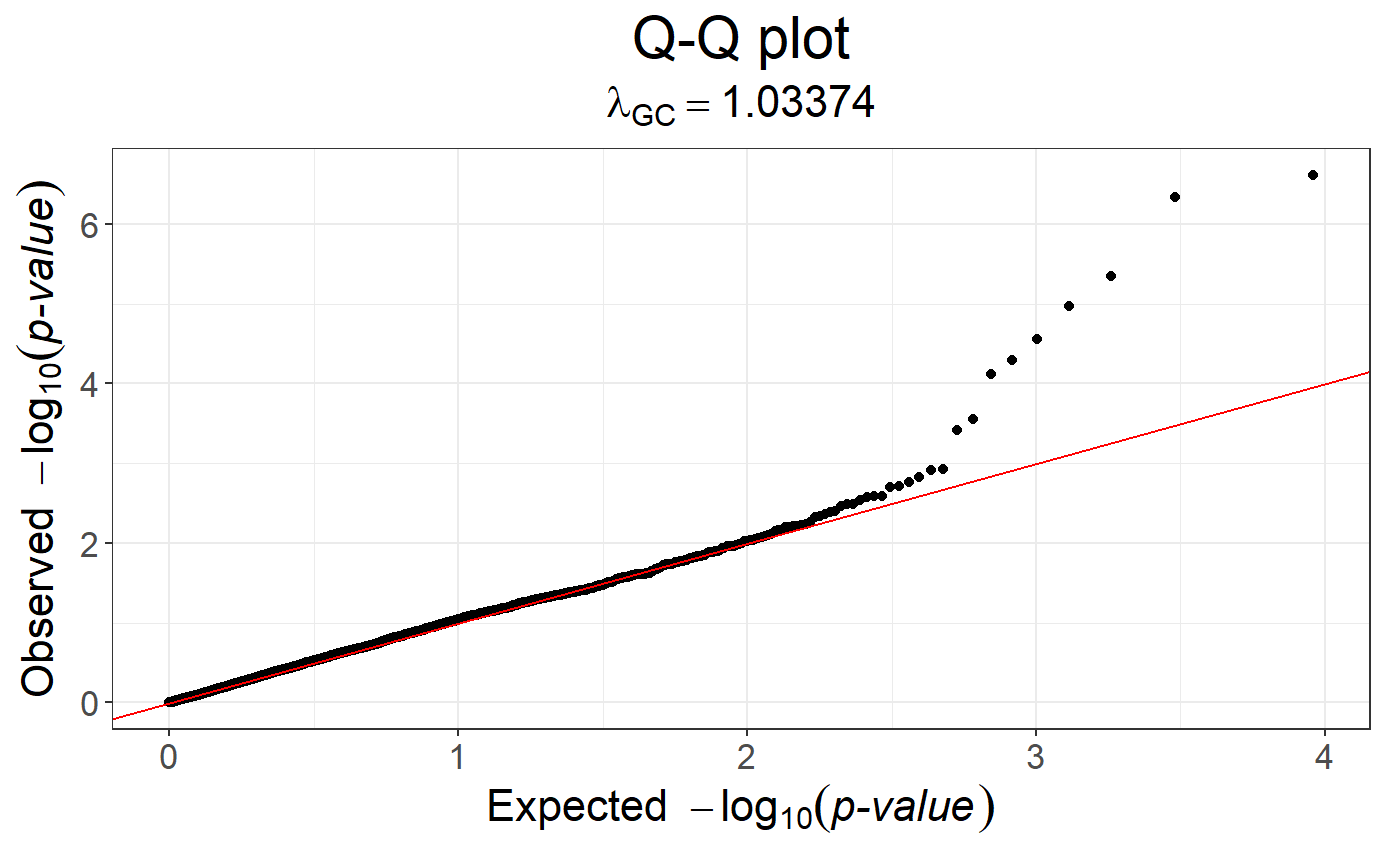# You can easily apply genomic control to this object obj.gwas.gc <- snp_gc(obj.gwas) # Redo the Q-Q plot snp_qq(obj.gwas.gc)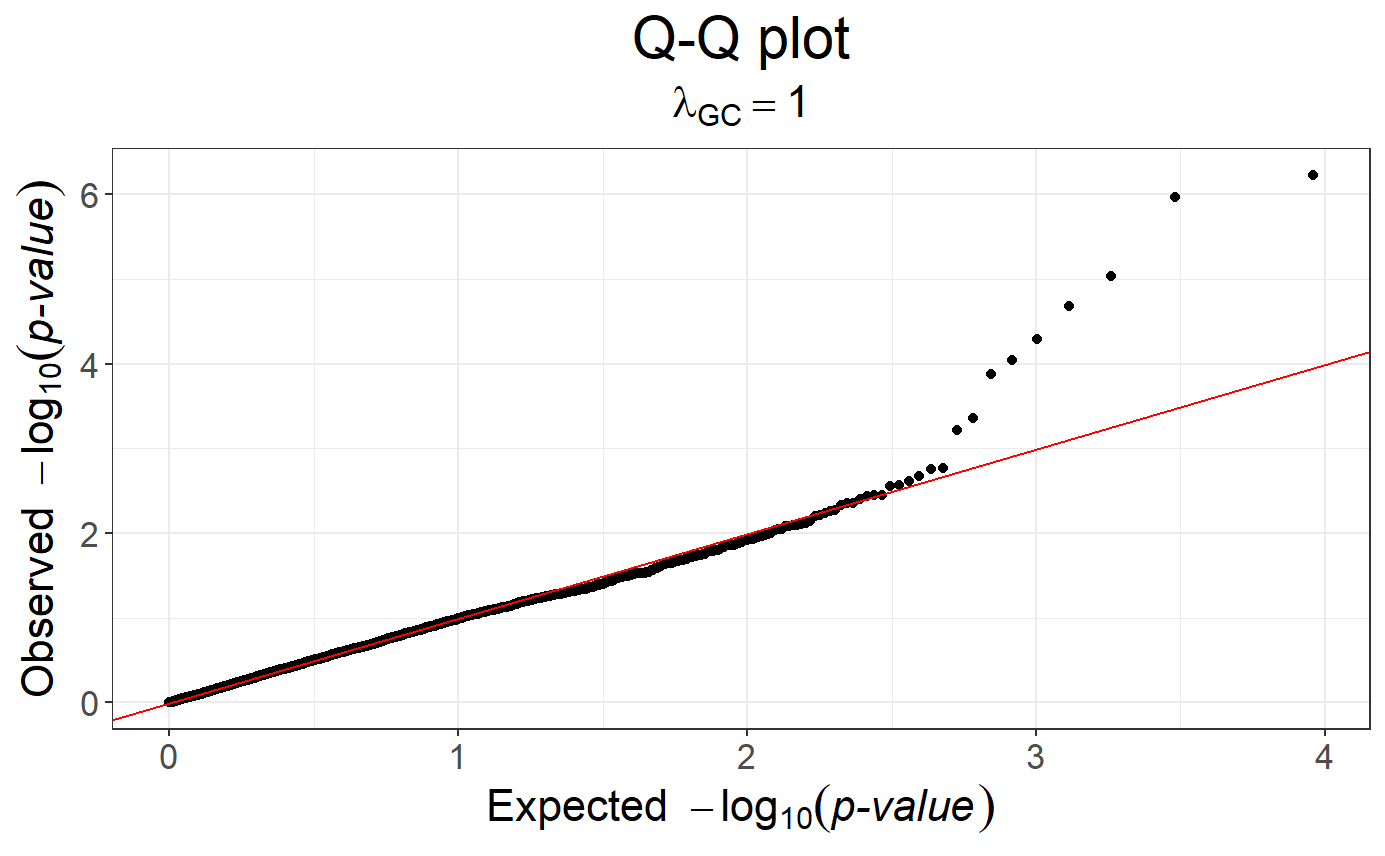# Manhattan plot, not quite sexy because there are only 1 chromosome here snp_manhattan(obj.gwas.gc, infos.chr = CHR, infos.pos = POS)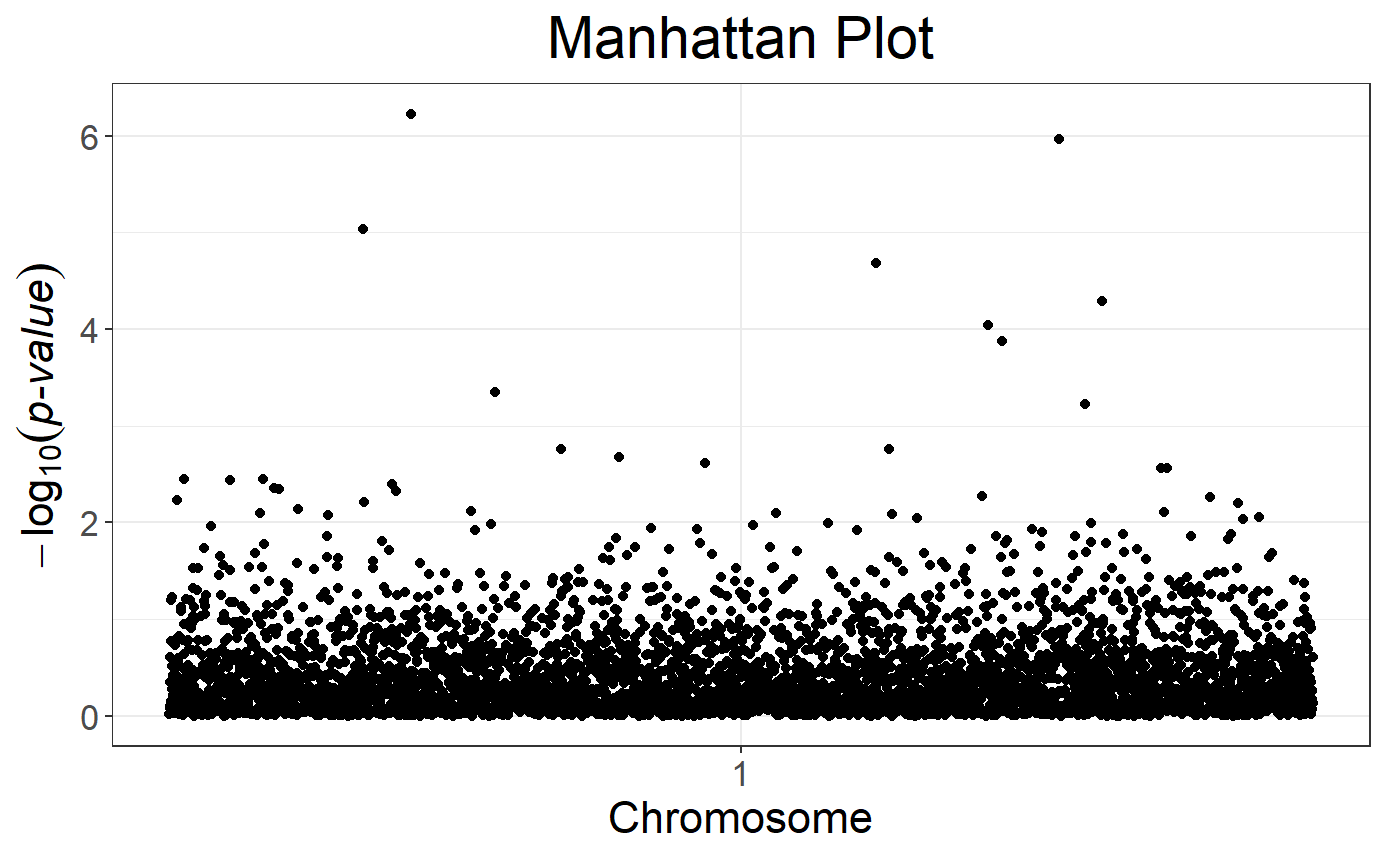## Joint PRS # Divide the indices in training/test sets ind.train <- sample(nrow(G), 400) ind.test <- setdiff(rows_along(G), ind.train) # Train the model cmsa.logit <- big_spLogReg(X = G, y01.train = y01[ind.train], ind.train = ind.train, covar.train = obj.svd$u[ind.train, ],
alphas = c(1, 0.5, 0.05, 0.001),
ncores = NCORES)
# Get predictions for the test set
preds <- predict(cmsa.logit, X = G, ind.row = ind.test,
covar.row = obj.svd\$u[ind.test, ])
# Check AUC
AUC(preds, y01[ind.test])
##  0.7113997